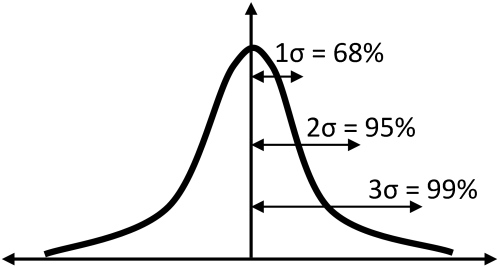# Example Code

• NI USB-568x

## Description

When performing radio frequency (RF) measurements, it is important to accurately estimate the uncertainty of the measurement. In this document, we show how to calculate the total (or combined) uncertainty of NI USB 568x RF power sensor measurements.

Absolute uncertainty percentages are not included in the specifications documents of NI USB 568x RF power sensors, since many of the factors are dependent on the sourcing instrument. Moreover, we need to follow a careful procedure to calculate the combined uncertainty from the individual uncertainties, which you can find in the specifications. On this web page, we include the step-by-step process to calculate the combined uncertainty, a numeric example, and an application that will do these calculations for you with the numeric inputs you specify.

To learn more about the types of uncertainties, refer to the National Institute of Standards and Technology (NIST) reference, below.

## How to Use

### 1. Calculating Individual Uncertainties

The first step in calculating the combined uncertainty is to calculate the individual uncertainties (refer to the specifications document for these values). To give a numeric example using the NI USB-5680, we will use a power level of 10 dBm and frequency of 2 GHz. Given these values, we can get the following uncertainty quantities from the specifications document:

 Uncertainty Value Definition and Comments Linearity 0.13 dB Uncertainty over the dynamic range of the digitization. Calibration factor 0.06 dB Uncertainty due to internal calibration constants. Noise 0.6 µW Uncertainty due to the effect of noise in the measurement. Zero set 1.7 µW Uncertainty due to the reference of “zero.” Zero the sensor for most accurate readings. Zero drift 0.5 µW Uncertainty due to drift of the actual zero. Temperature compensation 0.06 dB System uncertainty due to current temperature Effect of digital modulation 0.02 dB Uncertainty due to dynamic signals such as modulated waveforms. Mismatch Depends on source voltage standing wave ratio (VSWR) Uncertainty due to impedance mismatch between the NI USB-5680 and the device under test (DUT).

Table 1

Linearity and mismatch contribute the most to the combined uncertainty at higher powers, while at lower powers, noise, zero set and zero drift are also very important. To convert noise power to a percentage, divide the specification by the actual input power: 10 dBm or 0.01 W, in this example.

At higher power levels, noise is not be a major contributor to power accuracy. For reference, the table below illustrates the effect of noise, zero set, and zero drift at two distinct power levels. These numbers account for a coverage factor K=2. More details about coverage factor are explained below.

 Specification 0.01 W (10 dBm) 0.000001 W (–30 dBm) Noise 0.003% 0.125% Zero Set 0.0098 % 0.577 % Zero Drift 0.0025 % 0.15 %

Table 2

You can calculate mismatch uncertainty using a combination of both the source VSWR and the instrument VSWR. While mismatch uncertainty varies according to the DUT, let's assume that our DUT has a VSWR of 1.3. We also know from the specifications document that the NI USB-5680 has a VSWR of 1.2 at 2 GHz. Therefore:

Solving for Γ in the equation VSWR = (1-Γ)/(1+Γ)

ΓSensor = –0.0909

ΓSource = –0.1304

Mismatch (%) = 100 x [(1 ± (|ΓSensor| x |ΓSource|))² - 1] = 2.3856%

We will now convert all dB units to percentage units:

% = (10^(dB/10) – 1 ) x 100

The important concept here is to convert all uncertainties into statistical variances. To do this, we need to know what type of probability distribution function each uncertainty has. The following table summarize each uncertainty, the type of probability distribution the uncertainty follows, and the divisor that can be used to convert the uncertainty to one variance.

 Uncertainty term Uncertainty specification (%) Probability distribution Divisor Adjusted uncertainty (%) Linearity 3.038 Rectangular √3 1.8 Calibration factor 1.391 Normal (2σ) 2 0.7 Noise 0.006 Normal (2σ) 2 0.0 Zero set 0.017 Rectangular √3 0.0 Zero drift 0.005 Normal (2σ) 2 0.0 Temperature compensation N/A Rectangular √3 0.0 Effect of digital modulation N/A Rectangular √3 0.0 Mismatch 2.38 U-Shape √2 1.7

Table 3

### 2. Calculating Combined Standard Uncertainty

The combined standard uncertainty is defined by combining all the individual uncertainties in the following way:

Combined uncertainty (σ) = √(∑σ2)

Combined uncertainty is the square root of the sum of square of standard deviations. In our example, the combined uncertainty is σ=2.53%

### 3. Expanded Uncertainty

The final step is calculating the expanded uncertainty. This value is the product of the combined uncertainty and the coverage factor (k😞

Expanded Uncertainty = kσ

The value of k defines the confidence of the measurement. With a normal (Gaussian) distribution, a value k = 2 defines a confidence value of 95%, meaning 95% of measurements fall within ±kσ of the average value.

A value k = 3 defines 99%.Figure 1

National Instruments uses k = 2 in the NI USB-568x specifications.